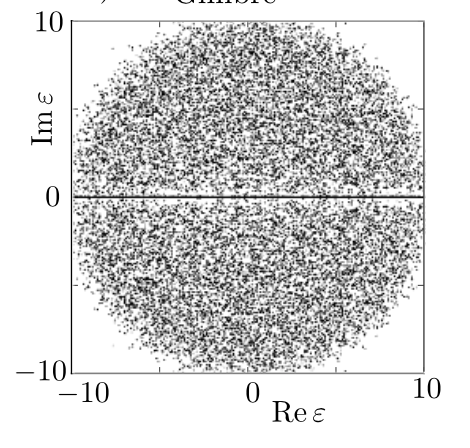# Purely complex eigenvalue of matrix product

Here is a question which arises from physics.

Let $A$, $B$ be two symmetric real-valued matrices. What conditions should the matrices meet to make $AB$ has a pure complex eigenvalue ($Im(\lambda) \neq 0$)?

Although I'm interested in complex eigenvalues it might be useful to see when $AB$ has real spectrum.

For instance, if $A$ is a positive form, then the spectrum of $AB$ is real-valued. Or if $[A,B] = 0$ then again spectrum of product is real. So there is no way to find a purely complex eigenvalue and we are done. What will happen if $A$ isn't positive definite? I'm searching for useful criteria that doesn't require positivity. Or maybe there exist criteria which ensure that $AB$ must have a purely complex eigenvalue.

UPD For instance, if matrices are 2x2, the criteria is the following:

$AB$ has only real eigenvalues $\Leftrightarrow$ $\exists$ a linear combination $\lambda A + \mu B^{-1}$ with real ${\lambda, \mu}$, s.t. it is positively defined

• if $A$ and $B$ are random $N\times N$ real matrices, you can expect the product $AB$ to have $\sqrt N$ real eigenvalues; the others will come in complex conjugate pairs off the real axis; this is not the deterministic criterium you ask for, which I don't think exists. Sep 16, 2017 at 11:41
• @CarloBeenakker why is it so? Sep 16, 2017 at 12:11
• @CarloBeenakker Does your result hold for symmetric $A$, $B$? The question is about symmetric matrices, but you don't write "symmetric" anywhere in your comment and answer. Asking just to be sure you didn't miss that part. Sep 16, 2017 at 15:33
• Converse: It is known that any real matrix can be written as the product of two real symmetric matrices -- this makes things "easy" speaking generically. Sep 16, 2017 at 17:17
• @FedericoPoloni --- some evidence for the large-$N$ universality of the eigenvalue density of the product of two random $N\times N$ matrices is given in arXiv:0912.3422. Sep 17, 2017 at 7:12

This is an intuitive argument for the $\sqrt N$-law, that a random real $N\times N$ matrix will have on average $\sqrt N$ eigenvalues on the real axis. The plot shows a scatter plot of the eigenvalues $\epsilon$ of 200 randomly generated $100\times 100$ matrices, for the case that the matrix elements have an independent normal distribution. As is common for random matrices, a different distribution will give similar results. (Several distributions are compared in Appendix A of arXiv:1305.2924.)If we rescale the eigenvalues so that their density in the complex plane is unity, then they will occupy an area of dimensions $\sqrt N\times\sqrt N$. As you can see, the density is uniform except near the real axis, where there is a depletion zone of area $1\times\sqrt N$.The $\sqrt N$ eigenvalues in that area condense onto the real axis.

This eigenvalue condensation happens because the eigenvalues of a real matrix come in complex conjugate pairs, so real eigenvalues are stable: a small perturbation cannot drive them off the real axis.

A mathematical way to describe the eigenvalue condensation is as a random walk on the real axis where pairs of random walkers vanish if they collide ("vicious" random walkers). This gives for the normal distribution the precise answer for the average number of real eigenvalues:

$$E(N_{\rm real})=\sqrt{2N/\pi}+1/2+{\cal O}(N^{-1/2})$$

As you can see in the cited paper (figure 8), other distributions have other coefficients, but the same $\sqrt N$ scaling.

The question is whether the $\sqrt{N}$ law holds for the product of two GOE matrices (two real symmetric matrices with Gaussian elements). I searched a bit in the literature, and found one paper that studies the product of two GUE matrices (two complex Hermitian matrices with Gaussian elements): Spectrum of the Product of Independent Random Gaussian Matrices. Because the eigenvalues of the product $XY$ of Hermitian X,Y still come in complex conjugate pairs, the same mechanism as for real matrices applies and the $\sqrt{N}$ law holds.

Really a remark on Carlo's answer, but somewhat relevant to the OP as well: if you generate random matrices as random products (that is, pick some matrices $A_1, \dotsc, A_N,$ and look at long random products), then the eigenvalues of the products will be almost surely all real. Why? Because the top eigenvalue will a unique eigenvalue of its modulus (this is a proximality statement, which you can find in the reference), and then a similar argument but with your product acting on the second exterior power shows that the biggest sum of two eigenvalues is real, and so on. What is also interesting is that the Galois group of the characteristic polynomial of this long random product (let's assume that the $A_i$ have integer entries) is $S_d.$

Breuillard, Emmanuel; Gelander, Tsachik, A topological Tits alternative., Ann. Math. (2) 166, No. 2, 427-474 (2007). ZBL1149.20039.

• Thanks, an explicit calculation by Forrester might be of interest in this connection: arxiv.org/abs/1309.7736 Sep 16, 2017 at 20:40
• @CarloBeenakker Thanks! That looks like a cool reference. Sep 17, 2017 at 21:37